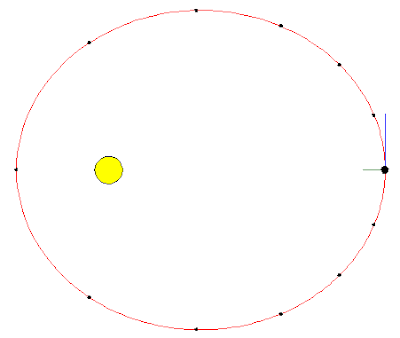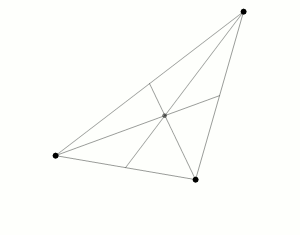### Three Books, Three Bodies, and No Solution

What I love about writing Astronomical Returns is that with nothing more than an article, a Wikipedia page, and a work of fiction, a mere ex-investment banker can share orbital mechanics with the world!
_______________________________________________________________

Since leaving investment banking and moving to LA and SpaceX, I find myself with a lot more free time, time which I'm trying to channel into productive pursuits like reading more books (and writing more Astronomical Returns articles for you!). A close friend of mine suggested The Three-Body Problem, and it's turned out to be an awesome trilogy that I highly recommend! I'm more of a nonfiction kind of reader; it's the first fiction book I've read since The Martian 5 years ago, which really goes to show how much I've enjoyed itOriginally written in Mandarin by Chinese author Cixin Liu, the trilogy has been expertly translated into English and is readily available

NO SPOILERS I promise, I myself just started the second book this week. But I wanted to feature it because the central plot element of the series revolves around the unsolvable three-body problem, a computational dilemma identified by none other than Isaac Newton and known in the field of astrodynamics for centuries! But how can a math problem possibly unsolvable - all the math we ever attempted in school always had an answer, right?? Well, many brilliant mathematicians have tried and failed to find an analytical solution, and while their work is far beyond my comprehension, I find it a worthwhile intellectual endeavor to at least understand the nature of the problemThe two-body problem is solvable - the motion will always take the form on an ellipse

Here it is in layperson's terms: it's of great practical value for us to be able to predict the motions of celestial bodies, based on present position and velocity. For example, if my satellite is currently over New York flying at 17,500 mph, how long until it's over Moscow so I can spy on the Soviets? Or if today is July 16, 1969 and Apollo 11 is on the launchpad, I better know exactly where the moon is going to be in four day's time! Turns out, if there are only two bodies in consideration, then we can predict the gravitational system exactly - Newton himself proved that the motion would take the form of an ellipse (see below for the solution to the two-body problem using Newton's Law of Universal Gravitation!!)Wow, that got wacky fast! Animation of three gravitationally-interacting bodies from Wikipedia - notice how the center of mass remains static

But in reality, we know considering only two bodies is a gross oversimplification, especially given that gravity works over infinite distances. Sure, the moon may orbit the Earth, but that doesn't mean gravity from the Sun, Jupiter, or any other body isn't distorting the moon's otherwise perfectly elliptical path. So then what if we took it just one tiny step further, and tried to predict the motions of three bodies? Surely we can do that, with all our intellect and computational power? The problem though, is that solving the two-body problem is done by assuming one body is "stationary" and just measuring the relative motion of the other body. For example, everyone knows both the Earth and the moon are hurtling through space, but if you're only concerned with the motion of the moon orbiting the Earth, then the Earth may as well be stationary! But as soon as you add the third body, there's no longer a fixed point relative to the other bodies, and determining a perfect solution is apparently no longer viable! (again, more mathematical explanation below)There are a few special cases of solvable three-body problems, like this figure 8 example (also from Wikipedia), but these are rather contrived and not particularly useful

Yikes! It appears math and science have utterly failed us. But if that's the case, and we can't even model the motions of three bodies, then how on Earth did Neil, Buzz, and Mike ever make it back from the moon? The answer lies in the relative magnitudes of the gravitational forces, and the approximations we can accordingly make. While it's true a lunar voyage should also consider the Sun's gravity, the Sun is far enough away that the force is orders of magnitude lower than what the Earth exerts on the moon, and everything else is utterly minuscule. From there, we can use numerical approximations to get us within an extremely precise range of where the moon is likely to beAnd this, my friends, is why NASA needs those big honking supercomputers!

So while we can't solve the three-body problem, we can usually get pretty close. Does this seem sketchy? Well the reality is, many of our advanced sciences are nothing more than highly precise approximations. For instance, the whole field of quantum mechanics never really solves anything, it's just a massive approximation that's so unbelievably precise it's good for all practical purposes. If "close enough" was fine by the crew of Apollo 11, then it's more than fine by me!

#### A little more detail...

The math here may seem a bit much, but I've included it because it provides way more insight as to why the three-body problem is so unsolvable than mere words ever could. It's adapted (i.e. further simplified) from this article here

Let's consider the two-body problem. The two formulas that are our starting point are Newton's 2nd law of motion, which states that force = mass * acceleration, and Newton's Law of Universal Gravitation, which computes gravitational force as a function of the two bodies' masses and the distance between them (recall that G is the universal gravitational constant)

$F = MA$
$F = \frac{GM_1M_2}{r^2}$

Assume there are two bodies with masses $M_1$ and $M_2$ with starting positions $\vec{P_1}$ and $\vec{P_2}$. Notice how in the two equations above, the left side of both is force. This means that to solve the gravitational force on $M_1$ exerted by $M_2$ we can say:

$M_1A_1 = \frac{GM_1M_2}{r^2}$, which in turn can be rewritten as

$M_1\ddot{\vec{P}}_1 = -\frac{GM_1M_2}{|\vec{P_1}-\vec{P_2}|^3}(\vec{P_1}-\vec{P_2})$
because acceleration is the second derivative of position (the double dot notation) and the vector between $\vec{P_1}$ and $\vec{P_2}$ is the radius

But why does this matter? Aren't we trying to describe the relative acceleration between these two bodies? The expression we really need to be solving is

$\ddot{\vec{P}}_1 - \ddot{\vec{P}}_2$

Well it turns out, using the expression we defined above for $M_1\ddot{\vec{P}}_1$, and using Newton's 3rd law of motion (which states every action has an equal but opposite reaction) to provide us $M_2\ddot{\vec{P}}_2 = -M_1\ddot{\vec{P}}_1$, with some fancy algebra and substitutions, we can demonstrate

$\ddot{\vec{P}}_1 - \ddot{\vec{P}}_2 = -\frac{G(M_1+M_2)}{|\vec{P_1}-\vec{P_2}|^3}(\vec{P_1}-\vec{P_2})$

(If you want the steps, see the aforementioned link)

From here, just replace $\vec{P_1}-\vec{P_2}$ with  $\vec{x}$, and $M_1+M_2$ with $M$, and you get

$\ddot{\vec{x}} = -\frac{GM\vec{x}}{|\vec{x}|^3}$

This equation in fact is exactly what describes the motion of an elliptical orbit!

Okay, that was pretty convoluted and painful, sorry about that. But what's important for our purposes is not that you actually followed along in finding the solution, just that you're convinced that one exists. With me so far? Good, because we're not even at the hard part yet!

All that work we did for the two-body problem was just to describe the attraction between body 1 and body 2. If there are 3 bodies, there are 3 different attractions occurring; if there are 4 bodies, there are 6 attractions, and if there are $N$ bodies, there are $\frac{N(N-1)}{2}$ different attractions to consider!The horror, the horror! It's getting ugly, FAST!

To make matters worse, not only are there more attractions, but the attraction on each body is the sum of the attractions of all the other bodies (this is the simplest case). Mathematically speaking, this would be laid out as

$M_1\ddot{\vec{P}}_1 = -\frac{GM_1M_2}{|\vec{P_1}-\vec{P_2}|^3}(\vec{P_1}-\vec{P_2}) -\frac{GM_1M_3}{|\vec{P_1}-\vec{P_3}|^3}(\vec{P_1}-\vec{P_3})$
$M_2\ddot{\vec{P}}_2 = -\frac{GM_2M_1}{|\vec{P_2}-\vec{P_1}|^3}(\vec{P_2}-\vec{P_1}) -\frac{GM_2M_3}{|\vec{P_2}-\vec{P_3}|^3}(\vec{P_2}-\vec{P_3})$
$M_3\ddot{\vec{P}}_3 = -\frac{GM_3M_1}{|\vec{P_3}-\vec{P_1}|^3}(\vec{P_3}-\vec{P_1}) -\frac{GM_3M_2}{|\vec{P_3}-\vec{P_2}|^3}(\vec{P_3}-\vec{P_2})$

It becomes incredibly burdensome because there's no fixed point to be referencing, and thus far, no one has solved it yet. But if you think you've got an answer, step right up! Your Nobel Prize and/or Fields Medal awaits!